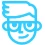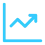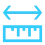Solved

# IIF - using account number

• 3 replies
• 45 views

Userlevel 3+1
• Freshman I
• 50 replies

Issue:  I need a formula to work one way when its to account 0500 and another way when it isn't.

I tried: 0500 ‘0500’ and “0500”, and the formula is only returning values for the false.

How to I format the expression to return the result for when its True.

= IIf( [GLTran.AccountID]= '0500', ( ([GLTran.CreditAmt] *.5) - ([GLTran.DebitAmt] ) *.5) , ([GLTran.CreditAmt] *.75) - ([GLTran.DebitAmt] ) *.75 )

icon

Best answer by aaghaei 20 April 2023, 21:58

View original

### 3 replies

Userlevel 7+4

Hi @jyounes! For this issue the GLTran.AccountID is actually not the AccountCD, this is a common misstep in creating calculations on ID fields.

Often the UI will switch the ID to the CD value so you can more easily understand what the value represents, but in this case the GLTran.AccountID is the numerical representation of the AccountCD, so it’s always false since you probably don’t have an account with the ID of 0500.

Try adding the =[GLTran.AccountID] field to the GI results to see the ID value of the account you’re looking to create the calculation on, then use this number instead of the 0500

Userlevel 7+8

The AccountID is in fact the system internal ID the way you use. You will need to Join GLTran with Account ON GLTran.AccountID = Account.AccountID and then use Account.AccountCD in your formula

Userlevel 3+1

The AccountID is in fact the system internal ID the way you use. You will need to Join GLTran with Account ON GLTran.AccountID = Account.AccountID and then use Account.AccountCD in your formula

Added the PX.Objects.GL.Account table and joined it the relations tab.  Added accountCD to the inquiry and referenced it in my formula and now I get the correct results.

UPDATED FORMULA

= IIf( [GLAccount.AccountCD] = '0500', ( ([GLTran.CreditAmt] *.5) - ([GLTran.DebitAmt] ) *.5) , ([GLTran.CreditAmt] *.75) - ([GLTran.DebitAmt] ) *.75 )